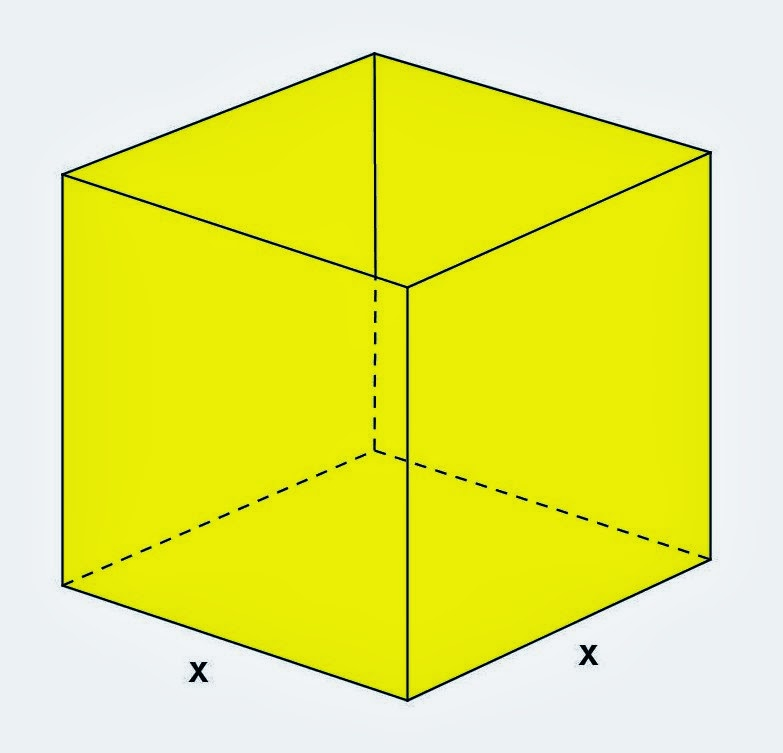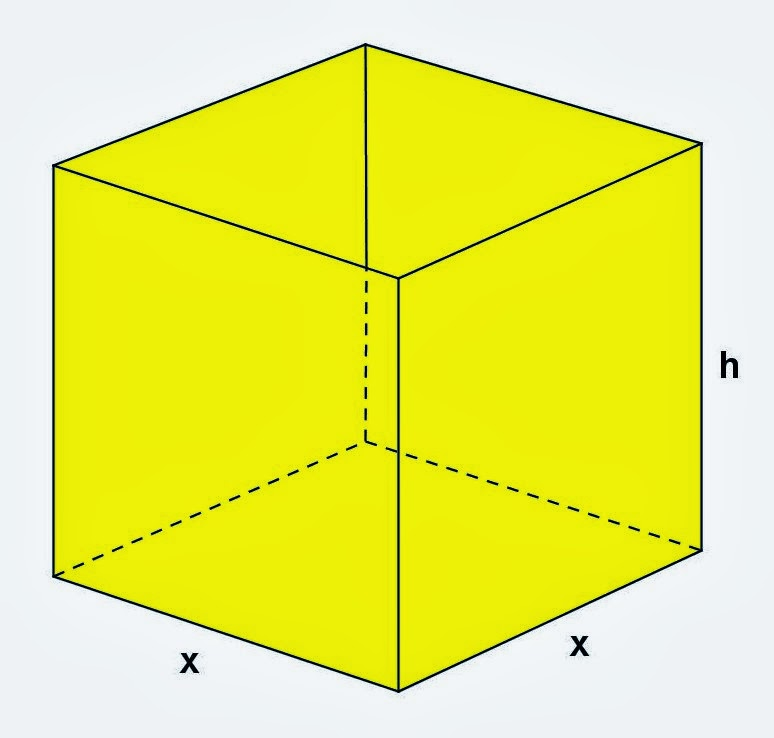## Tuesday, December 31, 2013

### Rectangular Parallelepiped Problem, 2

Category: Solid Geometry

"Published in Vacaville, California, USA"

A cardboard box has a square base, with each of the four edges of the base having length of x inches, as shown in the figure. The total length of all 12 edges of the box is 144 in.

(a) Express the volume V of the box as a function of x.
(b) Since both x and V represent positive quantities (length and volume, respectively), what is the domain of V?Photo by Math Principles in Everyday Life

Solution:

Consider the given figure abovePhoto by Math Principles in Everyday Life

The length of the square base of side x is given in the problem but the height of the rectangular box is not given. Let's assign h as the height of the rectangular box.Photo by Math Principles in Everyday Life

We know that the volume of a rectangular parallelepiped is

Since the base of a rectangular box is a square, then it follows that L = W = x. The height of the rectangular box is not given in the problem. If the perimeter or the total length of the sides of a rectangular box is given, then we can get the value of h which is the height as follows

(a) Therefore, the volume of a rectangular parallelepiped is

(b) The volume of a rectangular parallelepiped can be written as

If you will examine the above equation, we can assign x = 0 up to x = 18. Obviously, we cannot have a negative value of x which is the length of the sides of a rectangular box. Also, we cannot have x > 18 because the value of V will be negative. Therefore the domain of V will be equal to